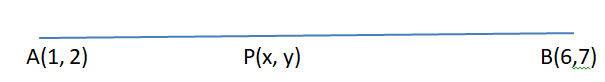Courses
Courses for Kids
Free study material
Free LIVE classes
More# Find the coordinates of the point P on the line segment joining $A(1,2)$ and $B(6,7)$ such that ${\text{AP = }}\dfrac{2}{5}{\text{AB}}{\text{.}}$Verified
327.9k+ views
Hint-In order to solve such a question we will simply use a section formula which tells us the coordinates of the point which divides a given line segment into two parts such that their lengths are in the ratio m:n.
$\left[ {\dfrac{{m{x_2} + n{x_1}}}{{m + n}},\dfrac{{m{y_2} + n{y_1}}}{{m + n}}} \right]$
Where m, n is the ratios and x, y is the coordinates.As we know that section formula or required coordinates of the point is given as
$\left[ {\dfrac{{m{x_2} + n{x_1}}}{{m + n}},\dfrac{{m{y_2} + n{y_1}}}{{m + n}}} \right]$

Given that the coordinates of the line segment $A(1,2)$ and $B(6,7)$
And the relation as
${\text{AP = }}\dfrac{2}{5}{\text{AB}}{\text{.}}$
Let the coordinates of point be $P\left( {x,y} \right)$ then
Here, point P is on AB such that ${\text{AP = }}\dfrac{2}{5}{\text{AB}}{\text{.}}$
$\Rightarrow \dfrac{{{\text{AP}}}}{{{\text{AB}}}} = \dfrac{2}{5} \\ \Rightarrow 5{\text{AP = 2AB }}\left[ {\because {\text{ AB = AP + PB}}} \right] \\ \Rightarrow {\text{5AP = 2(AP + PB)}} \\ \Rightarrow {\text{5AP = 2AP + 2PB}} \\ \Rightarrow {\text{3AP = 2PB}} \\ \Rightarrow \dfrac{{{\text{AP}}}}{{{\text{PB}}}} = \dfrac{2}{3} \\$
This means P divides AB in the ratio 2:3

As, we know that the section formula for required coordinate of the point is given as $\Rightarrow \left( {\dfrac{{{m_2} + n{x_1}}}{{m + n}},\dfrac{{m{y_2} + n{y_1}}}{{m + n}}} \right)$
The ratio in which point P divides the line is 2:3,
Thus m=2, n=3
And the line points coordinates are $A(1,2)$ and $B(6,7)$
Therefore coordinates of P will be
$\Rightarrow \left( {\dfrac{{{m_2} + n{x_1}}}{{m + n}},\dfrac{{m{y_2} + n{y_1}}}{{m + n}}} \right) \\ \Rightarrow \left( {\dfrac{{2 \times 6 + 3 \times 1}}{{2 + 3}},\dfrac{{2 \times 7 + 3 \times 2}}{{2 + 3}}} \right) \\ \Rightarrow \left( {\dfrac{{15}}{5},\dfrac{{20}}{5}} \right) \\ \Rightarrow (3,4) \\$

Hence, the coordinates of the point which divides the line segment joining $(1,2)$ and B $(6,7)$ internally in the ratio $2:3$ is $\left( {3,4} \right)$.

Note- To solve these types of problems remember all the formulas of coordinate geometry. And try to draw a rough sketch of the diagram on the paper, this helps a lot in solving the question. This problem can also be done by graphical method but coordinate geometry method is always the easiest and less time consuming method.

Last updated date: 02nd Jun 2023
Total views: 327.9k
Views today: 5.84k